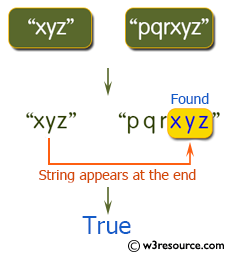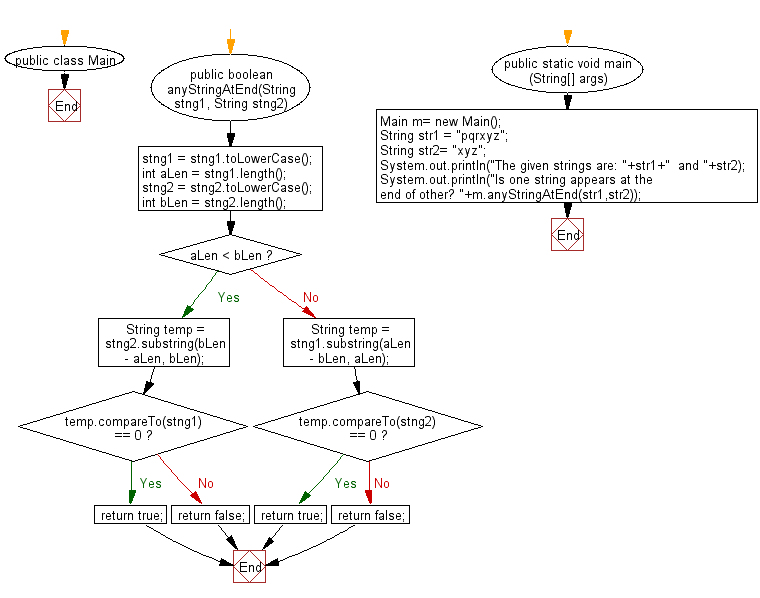﻿ Java exercises: Check two given strings whether any one of them appear at the end of the other string - w3resource# Java String Exercises: Check two given strings whether any one of them appear at the end of the other string

## Java String: Exercise-71 with Solution

Write a Java program to check two given strings whether any one of them appear at the end of the other string (ignore case sensitivity).

Sample Solution:

Java Code:

``````import java.util.*;
public class Main
{
public boolean anyStringAtEnd(String stng1, String stng2)
{
stng1 = stng1.toLowerCase();
int aLen = stng1.length();
stng2 = stng2.toLowerCase();
int bLen = stng2.length();
if (aLen < bLen)
{
String temp = stng2.substring(bLen - aLen, bLen);
if (temp.compareTo(stng1) == 0)
return true;
else
return false;
} else
{
String temp = stng1.substring(aLen - bLen, aLen);
if (temp.compareTo(stng2) == 0)
return true;
else
return false;
}
}
public static void main (String[] args)
{
Main m= new Main();
String str1 =  "pqrxyz";
String str2= "xyz";
System.out.println("The given strings are: "+str1+"  and "+str2);
System.out.println("Is one string appears at the end of other? "+m.anyStringAtEnd(str1,str2));
}
}
``````

Sample Output:

```The given strings are: xyz  and pqrxyz
Is one string appears at the end of other? true

The given strings are: pqrxyz  and xyz
Is one string appears at the end of other? true
```

Pictorial Presentation:Flowchart:Java Code Editor:

Improve this sample solution and post your code through Disqus

What is the difficulty level of this exercise?

﻿

## Java: Tips of the Day

Parsing dates:

```import java.io.*;
import java.util.*;
import java.text.*;

String s = "2001/09/23 14:39";

SimpleDateFormat formatter = new SimpleDateFormat ("yyyy/MM/dd H:mm");
Date d = formatter.parse(s, new ParsePosition(0));
```